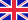•Elastic properties and Equations of State

Autor: Sushil Kumar Pathak

Phenomenological equations represent the relationship between pressure (P) and volume (V) which can expressed analytically involving two parameters and which are respectively, the isothermal bulk modulus and its pressure derivative both are zero pressure.... Viac o knihe

Na objednávku, dodanie 2-4 týždne

31.45 €

bežná cena: 37.00 €

O knihe

Phenomenological equations represent the relationship between pressure (P) and volume (V) which can expressed analytically involving two parameters and which are respectively, the isothermal bulk modulus and its pressure derivative both are zero pressure. This is not always the case for equation of state derived from interionic potentials using some specific potential function for the short range potential energy. For example, the Born-Mayer gives an expotential potential function for the short range potential energy. Equation of state where is a complicated expression involving potential parameters.

• Vydavateľstvo: LAP LAMBERT Academic Publishing
• Rok vydania: 2018
• Formát: Paperback
• Rozmer: 220 x 150 mm
• Jazyk: Anglický jazyk
• ISBN: 9786138144427

Generuje redakčný systém BUXUS CMS spoločnosti ui42.